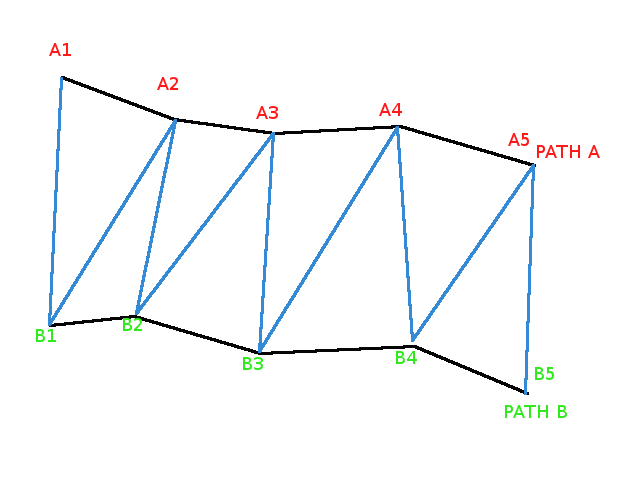# Ribbon In Detail

## Ribbon in Detail

Lets take a closer look at a ribbon

It is formed using paths. A path is simply an array of a minimum of two vector3 points.

Below shows the construction of a ribbon using two paths A and B each having five points.Here's a simple example:

Simple Ribbon Example

You can have as many paths as you wish, including just one, you can close all the paths and/or close the paths array itself. Imagine a long ribbon of narrow width in the real world with wire running down its length. Closing the paths forms a loop of ribbon while closing the array would form a tube.

## Single Path Ribbon

First construct the single path, for example

pathHelix = [];let v;for (let i = 0; i <= 60; i++) {  v = (2.0 * Math.PI * i) / 20;  pathHelix.push(new BABYLON.Vector3(3 * Math.cos(v), i / 4, 3 * Math.sin(v)));}

Showing the path: Showing Ribbon Path

The ribbon is formed by joining each point on the path to a later point where one exists. The offset property governs how many points ahead the current point with be joined to. The triangular facets for the mesh are formed from the current point, the next point and the offset point.

For point p and offset f the triangle is p, p + 1, p + f, provided of course that p + f < number of points in the path array

Create the ribbon with a variety of offsets and show in wireframe
default offset, half the path length (60 / 2 = 30): Create a Ribbon Example 1 offset 10: Create a Ribbon Example 2 offset 5: Create a Ribbon Example 3 offset 20: Create a Ribbon Example 4

So playing with offset, closeArray, or other parameters, you can easily get volumes, even with a single path: Create a Ribbon Example 5

## Length of Paths

It's not mandatory that all the ribbon paths have the same length, but it is not recommended.
The best way to emulate different lengths for some parts of your mesh is then to simply use many ribbons.

In this example: Create a Ribbon With Path Lengths path2 and path3 are longer than path1 and path4.

As you can see, the final ribbon adjusts to different lengths. The rule is they all start from first path points and each intermediate ribbon then stops at first of its both constituting paths end. However, while you can add color using a material, as done here: Create a Colored Ribbon

There is no incidence on light reflection for ribbon with different length paths. Therefore you can't add a texture to a ribbon constructed with different length paths.
This is due to the fact that the nested ribbon texturing algorithm only knows how to deal with a unique length for all paths. When applying a texture the algorithm assumes that the ribbon can be unfolded into a rectangle that can stretched to fit on top of the image used for the texture. This is only possible when paths are of equal length.

## Closed shapes : normals or textures ?

The ribbon mesh provides two ways to automatically close an unclosed shape.

• closeArray parameter : this will add an extra unit ribbon between the last path and the first path of your pathArray.
• closePath parameter : this will join the last and first points of each path in your pathArray.
var ribbon = BABYLON.MeshBuilder.CreateRibbon("ribbon", { pathArray: paths }, scene);
var ribbon = BABYLON.MeshBuilder.CreateRibbon("ribbon", { pathArray: paths, closeArray: true }, scene);
paths.push(paths);var ribbon = BABYLON.MeshBuilder.CreateRibbon("ribbon", { pathArray: paths }, scene);